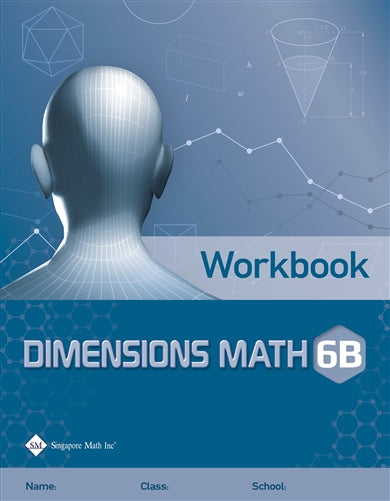# Singapore Math Dimensions Math Workbook 6B

Workbooks are the essential supplements to textbooks as they give students the opportunity to practice applying new concepts. Workbook exercises polish analytical skills and help students develop a stronger foundation in math through repetition and variation.

Features & Components:

• Basic Practice: Simple questions that drill comprehension of concepts.
• Further Practice: More difficult questions that involve direct application.
• Challenging Practice: Questions that require synthesis.

The answer key at the back of the book provides answers only. Workbook Solutions (sold separately) contain fully worked solutions.

Note: Two workbooks (A and B) correspond to the two halves of the school year. Soft cover.

Dimensions Math® Workbook 6B

Chapter 8: Algebraic Expressions

8.1 Writing and Evaluating Algebraic Expressions

A. Use of Letters
B. Evaluating Algebraic Expressions
C. Word Problems

8.2 Simplifying Algebraic Expressions

In A Nutshell

Chapter 9: Equations and Inequalities

9.1 Equations

A. Algebraic Equations
B. Balancing Equations

9.2 Inequalities

A. Algebraic Inequalities
B. Graphing Inequalities Using a Number Line

In A Nutshell
Problem Solving Corner

Chapter 10: Coordinates and Graphs

10.1 The Coordinate Plane
10.2 Distance between Coordinate Pairs
10.3 Changes in Quantities

A. Independent and Dependent Variables
B. Representing Relationships between Variables
C. Observing Relations between Variables with Graphs

In A Nutshell

Chapter 11: Area of Plane Figures

11.1 Area of Rectangles and Parallelograms
11.2 Area of Triangles

A. Finding Area of a Triangle
B. Areas Involving Parallelograms and Triangles

11.3 Area of Trapezoids

In A Nutshell

Chapter 12: Volume and Surface Area of Solids

12.1 Volume of Rectangular Prisms

A. Cubes and Cuboids
B. Volume of Liquids

12.2 Surface Area of Prisms

A. Surface Area of Rectangular Prisms
B. Surface Area of Triangular Prisms
C. Metric Conversions and Volume

In A Nutshell
Problem Solving Corner

Chapter 13: Displaying and Comparing Data

13.1 Statistical Variability

A. Statistical Questions
B. Measures of Center

13.2 Displaying Numerical Data

A. Dot Plots
B. Histograms

13.3 Measures of Variability and Box Plots

A. Range
B. Mean Absolute Deviation
C. Interquartile Range
D. Box Plot

In A Nutshell